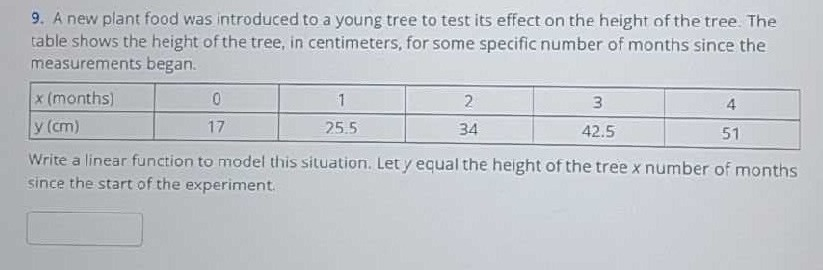### ¿Todavía tienes preguntas de matemáticas?

Pregunte a nuestros tutores expertos
Algebra
Pregunta9. A new plant food was introduced to a young tree to test its effect on the height of the tree. The table shows the height of the tree, in centimeters, for some specific number of months since the measurements began.

Write a linear function to model this situation. Let $$y$$ equal the height of the tree $$x$$ number of months since the start of the experiment.